# CHSE Odisha Class 11 Math Solutions Chapter 9 Binomial Theorem Ex 9(a)

Odisha State Board CHSE Odisha Class 11 Math Solutions Chapter 9 Binomial Theorem Ex 9(a) Textbook Exercise Questions and Answers.

## CHSE Odisha Class 11 Math Solutions Chapter 9 Binomial Theorem Exercise 9(a)

Question 1.
The rows n = 6 and n = 7 in the pascal triangle have been kept vacant. Fill in the gaps
Solution: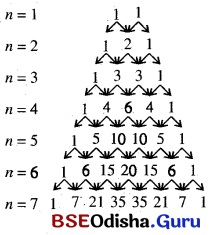Question 2.
Write down the expansion of (a + b)8 using Pascal’s triangle.
Solution:
The row n = 8 in Pascal’s triangle is 1, 8, 28, 56, 70, 56, 28, 8, 1.
∴ (a + b)8 = a8 + 8a8-1b1 + 28a8-2b2 + 56a8-3b3 + 70a8-4b4 + 56a8-5b5 + 28a8-6b6 + 8a8-7b7 + b8
= a8 + 8a7b + 28a6b2 + 56a5b3 + 70a4b4 + 56a3b5 + 28a2b6 + 8ab7 + b8

Question 3.
Find the 3rd term in the expansion of $$\left(2 x^3-\frac{1}{x^6}\right)^4$$ using rules of Pascal triangle.
Solution: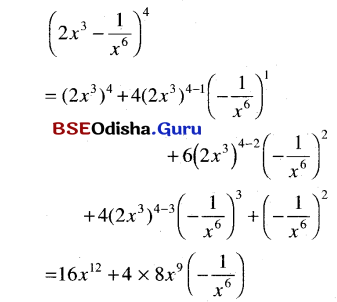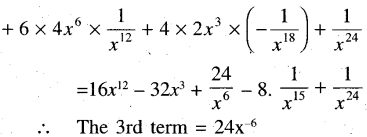Question 4.
Expand the following :
(a) (7a + 3b)6
Solution:
(7a + 3b)6 = (7a)6 + 6C1(7a)6-1(3b)1 + 6C2(7a)6-2(3b)2 + ….. + (3b)676a6 + 6(7a)5(3b) + 15(7a4) × 9b2 + …. + 36b6
= 7a6 + 18 × 75a5b + 135 × 74a4b2 + ….. + 36b6

(b) $$\left(\frac{-9}{2} a+b\right)^7$$
Solution: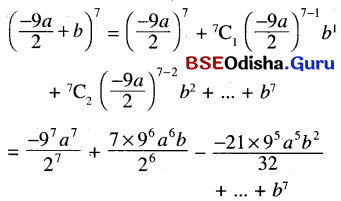(c) $$\left(a-\frac{7}{3} c\right)^4$$
Solution: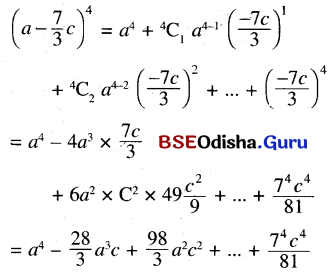Question 5.
Apply Binominal Theorem to find the value of (1.01)5.
Solution:
= 1 + 5C1(0.01)1 + 5C2(0.01)2 + 5C3(0.01)3 + 5C4(0.01)4 + (0.01)5
= 1 + 5 × 0.01 + 10(0.0001) + 10(0.000001) + 5(0.00000001) + 0.0000000001
= 1 + 0.05 + 0.001 + 0.00001 + 0.00000005 + 0.000000001
= 1.0510100501

Question 6.
State true or false.
(a) The number of terms in the expansion of $$\left(x^2-2+\frac{1}{x^2}\right)^6$$ is equal to 7.
Solution:
False

(b) There is a term independent of both x and y in the expansion of $$\left(x^2+\frac{1}{y^2}\right)^9$$
Solution:
False

(c) The highest power in the expansion of $$x^{40}\left(x^2+\frac{1}{x^2}\right)^{20}$$ is equal to 40.
Solution:
False

(d) The product of K consecutive natural numbers is divisible by K!
Solution:
TrueQuestion 7.
(a) If the 6th term in the expansion of (x + *)n is equal to nC5xn-10 find *
Solution:
Let the 6th term (x + y)n is nC5xn-10
nC5xn-5y5 = nC5xn-10 = nC5xn-5.x-5
⇒ y5 = x-5 = $$\frac{1}{x^5}$$
∴ y = $$\frac{1}{x}$$ . Hence * = $$\frac{1}{x}$$

(b) Find the number of terms in the expansion of (1 + x)n (1 – x)n.
Solution:
(1 + x)n (1 – x)n = (1 – x2)n
∴ The number of terms in this expansion is (n + 1)

(c) Find the value of $$\frac{{ }^n \mathrm{C}_{r-1}}{{ }^n \mathrm{C}_r}$$
Solution: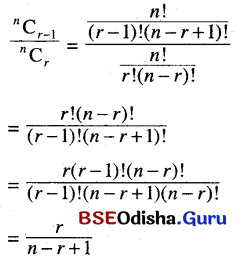(d) How many terms in the expansion of $$\left(\frac{3}{a}+\frac{a}{3}\right)^{10}$$ have positive powers of a? How many have negative powers of a?
Solution: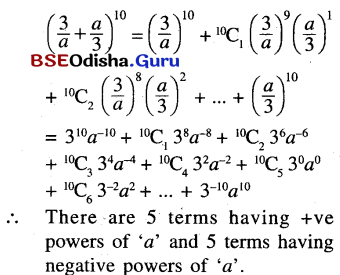Question 8.
Find the middle term(s) in the expansion of the following.
(a) $$\left(\frac{a}{b}+\frac{b}{a}\right)^6$$
Solution:
Here there is only one middle term i.e. the 4th term.
∴ 4th term i.e. (3 + 1)th term in the expansion of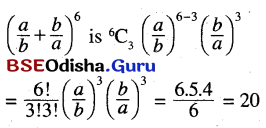(b) $$\left(x+\frac{1}{x}\right)^9$$
Solution:
Here there are two middle terms i.e. 5th and 6th terms.
∴ 5th term in the above expansion is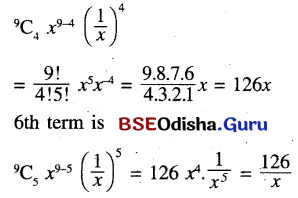(c) $$\left(x^{\frac{3}{2}}-y^{\frac{3}{2}}\right)^8$$
Solution:
Here there is only one middle term i.e. 5th term.
∴ 5th term i.e. (4 + 1)th term in the expansion of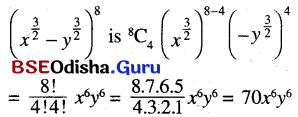Question 9.
Find the 6th term in the expansion of $$\left(x^2+\frac{a^4}{y^2}\right)^{10}$$
Solution:
6th term i.e. (5 + 1)th term in the expansion of
(x2 + $$\frac{a^4}{y^2}$$)10 is 10C5(x2)10-5 ($$\frac{a^4}{y^2}$$)5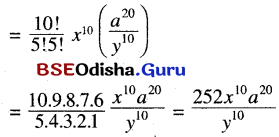Question 10.
(a) Find the fifth term in the expansion of (6x – $$\frac{a^3}{x}$$)10
Solution: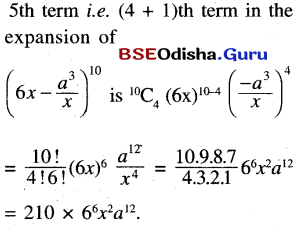(b) Is there a term independent of x? If yes find it out.
Solution:
Let (r + 1)th term in the expansion of  (6x – $$\frac{a^3}{x}$$)10 is independent of x.
∴ (r + 1)th term = 10Cr(6x)10-r($$\frac{-a^3}{x}$$)r
= 10Cr610-rx10-r(-1)ra3rx-r
= (-1)r 10Cr610-ra3rx10-2r
∴ x10-2r = 1 = x0
or, 10 – 2r = 0 or, r = 5
∴ 6th term is term independent of x in the above expansion and the term is (-1)5 10C5610-5a3.5
= – 10C565a15 = – 252 × 65a15Question 11.
(a) Find the coefficient of $$\frac{1}{y^{10}}$$ in the expansion of $$\left(y^3+\frac{a^7}{y^5}\right)^{10}$$
Solution: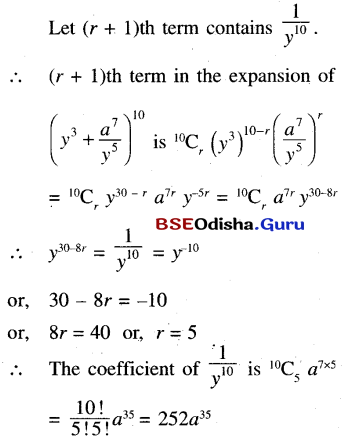(b) Does there exist a term independent of y in the above expansion?
Solution:
Let (r + 1)th term is independent of y.
∴ y30-8r = 1 = y0 or, 30 – 8r = 0
or, r = $$\frac{30}{8}$$ = $$\frac{15}{4}$$ which is not possible as r∈ N or zero.
∴ There is no term in the expansion of $$\left(y^3+\frac{a^7}{y^5}\right)^{10}$$ which is independent of y.

Question 12.
(a) Find the coefficient of x4 in the expansion of (1 + 3x + 10x2)(x + $$\frac{1}{x}$$)10
Solution: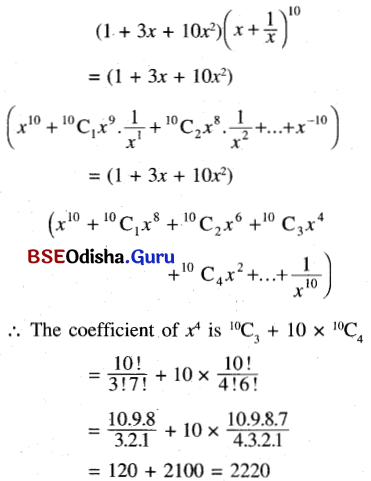(b) Find the term independent of x in the above expansion.
Solution: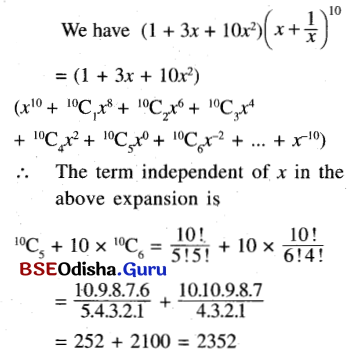Question 13.
Show that the coefficient of am and an in expansion of (1 + a)m+n are equal.
Solution:
(m + 1)th and (n + 1)th terms in the expansion of (1 + a)m+n are m+nCmam and m+nCnan
∴ The coefficient of am and an are m+nCm and m+nCn which are equal.Question 14.
An expression of the form (a + b + c + d + …. ) consisting of a sum of many distinct symbols called a multinomial. Show that (a + b + c)n is
the sum of all terms of the form $$\frac{\boldsymbol{n} !}{\boldsymbol{p} ! \boldsymbol{q} ! \boldsymbol{r} !}$$ apbqcr where p, q and r range over all positive triples of non-negative integers such that p + q + r = n.
Solution: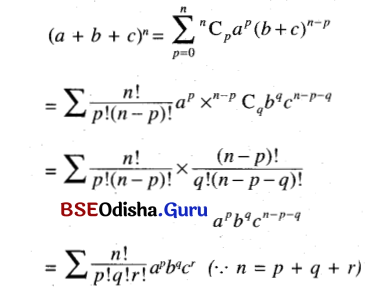Question 15.
State and prove a multinominal Theorem.
Solution:
Multinominal Theorem:
(P1 + P2 + ……. + Pm)n
$$=\sum \frac{n !}{n_{1} ! n_{2} ! \ldots n_{m} !} p_1^{n_1} p_2^{n_2} \ldots p_m^{n_m}$$
where n1 + n2 + ……. + nm = n
The proof of this theorem is beyond the syllabus.